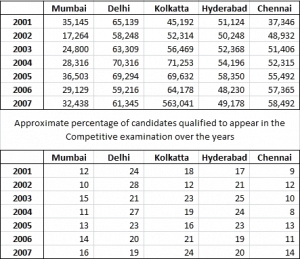# Data Interpretation Questions For SBI Clerk PDF Set-2

1
5317

Data Interpretation questions, will be asked in numerical ability section of SBI Clerk. The questions will be appeared both in prelims and mains exam. There will be around 2-3 Sets having total of 6-10 questions on DI (Data Interpretation). Hence we have given some sample DI Questions from previous asked papers.

# Data Interpretation Questions For SBI Clerk PDF Set-2

Instructions:

Study the following tables carefully and answer the questions given below:
Number of Candidates appeared in a Competitive Examination from five centres over the yearsQuestion 1:

In which of the following years was the difference in number of candidates appeared from Mumbai over the previous year the minimum ?

a) 2004
b) 2006
c) 2007
d) 2002
e)None of these

Question 2:

In which of the following years was the number of candidates qualified from Chennai, the maximum among the given years ?

a) 2007
b) 2006
c) 2005
d) 2003
e)None of these

Question 3:

Approximately what was the total number of candidates qualified from Delhi in 2002 and 2006 together ?

a) 27250
b) 25230
c) 30150
d) 28150
e)26250

Question 4:

Approximately how many candidates appearing from Kolkata in 2004 qualified in the competitive examination ?

a) 13230
b) 13540
c) 15130
d) 15400
e)19240

Question 5:

Approximately what was the difference between the number of candidates qualified from Hyderabad in 2001 and 2002 ?

a) 1680
b) 2440
c) 1450
d) 2060
e)1860

Solutions 1 to 5

The drastic fall of the number of candidates from 2001 (35,145) to 2002 (17,264) is 17,881.
In every other year (except a smaller drop from 2005-2006), the number of candidates has increased from one year to the next.
Hence, the correct answer is 2002

In 2007, the highest number of candidates appeared for the exam from Chennai and the pass percentage is also the highest compared to the other years. So, the number of candidates from Chennai who passed in the exam is the highest in 2007.
The number equals 14%*58492 = 8189